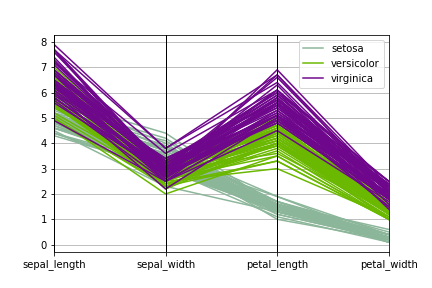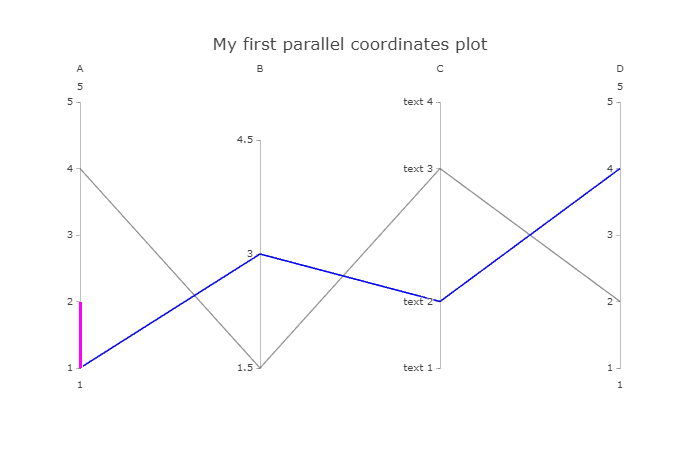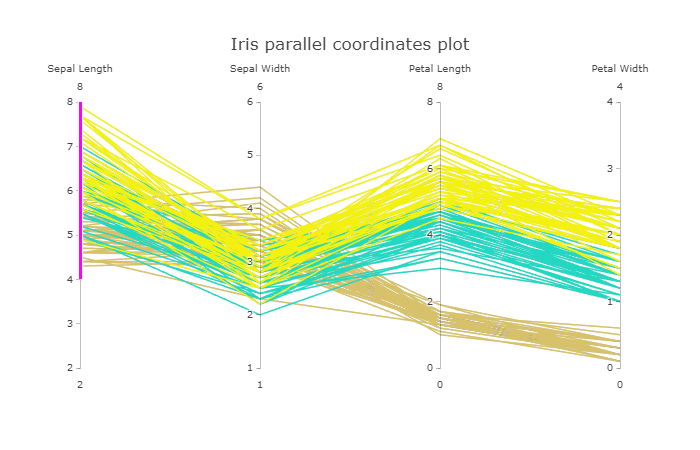• 平行坐标图简介当数据的维度超过三维时，此时数据的可视化就变得不再那么简单。为解决高维数据的可视化问题，我们可以使用平行坐标图。以下关于平行坐标图的解释引自百度百科：为了克服传统的笛卡尔直角坐标系容易...
平行坐标图简介
当数据的维度超过三维时，此时数据的可视化就变得不再那么简单。为解决高维数据的可视化问题，我们可以使用平行坐标图。以下关于平行坐标图的解释引自百度百科：为了克服传统的笛卡尔直角坐标系容易耗尽空间、 难以表达三维以上数据的问题， 平行坐标图将高维数据的各个变量用一系列相互平行的坐标轴表示， 变量值对应轴上位置。为了反映变化趋势和各个变量间相互关系，往往将描述不同变量的各点连接成折线。所以平行坐标图的实质是将m维欧式空间的一个点Xi(xi1,xi2,...,xim) 映射到二维平面上的一条曲线。在N条平行的线的背景下，（一般这N条线都竖直且等距），一个在高维空间的点可以被表示为一条拐点在N条平行坐标轴的折线，在第K个坐标轴上的位置就表示这个点在第K个维的值。
绘制平行坐标图
本文主要介绍两种利用Python绘制平行坐标图的方法，分别是利用pandas包绘制和利用plotly包绘制(默认已安装pandas包和plotly包)。
利用pandas实现平行坐标图的绘制
import matplotlib.pyplot as plt
import pandas as pd
import seaborn as sns
from pandas.plotting import parallel_coordinates
fig,axes = plt.subplots()
parallel_coordinates(data,'species',ax=axes)
fig.savefig('parallel.png')
绘制的平行坐标图如下所示：从上图可以看到x轴上变量共用一个y坐标轴，此时因sepal_length、sepal_width、petal_length以及petal_width这四个变量的值得范围相近，利用这种方式作出的共用y轴的平行坐标图有着很好的可视化效果；但假如sepal_length、sepal_width、petal_length以及petal_width这些变量的值的范围相差较大时，这种共用y轴的平行坐标图就不再适用，此时我们需要的是y轴独立的平行坐标图。下面介绍的另一种方法实现的就是y轴独立的平行坐标图。
利用plotly实现平行坐标图的绘制
plotly绘图有两种模式，一种是online模式，另一种是offline模式。本文使用的是offline模式，且是在jupyter notebook中进行绘图。
首先熟悉一下plotly的绘图方式：
import plotly as py
import plotly.graph_objs as go
py.offline.init_notebook_mode(connected=True) # 初始化设置
py.offline.iplot({
"data": [go.Parcoords(
line = dict(color = 'blue'),
dimensions = list([
dict(range = [1,5],
constraintrange = [1,2],
label = 'A', values = [1,4]),
dict(range = [1.5,5],
tickvals = [1.5,3,4.5],
label = 'B', values = [3,1.5]),
dict(range = [1,5],
tickvals = [1,2,4,5],
label = 'C', values = [2,4],
ticktext = ['text 1', 'text 2', 'text 3', 'text 4']),
dict(range = [1,5],
label = 'D', values = [4,2])
])
)],
"layout": go.Layout(title="My first parallel coordinates")
})
绘制图形如下所示：绘制鸢尾花数据的平行坐标图：
df['species_id'] = df['species'].map({'setosa':1,'versicolor':2,'virginica':3}) #用于颜色映射
py.offline.iplot({
"data": [go.Parcoords(
line = dict(color = df['species_id'],
colorscale = [[0,'#D7C16B'],[0.5,'#23D8C3'],[1,'#F3F10F']]),
dimensions = list([
dict(range = [2,8],
constraintrange = [4,8],
label = 'Sepal Length', values = df['sepal_length']),
dict(range = [1,6],
label = 'Sepal Width', values = df['sepal_width']),
dict(range = [0,8],
label = 'Petal Length', values = df['petal_length']),
dict(range = [0,4],
label = 'Petal Width', values = df['petal_width'])
])
)],
"layout": go.Layout(title='Iris parallel coordinates plot')
})
绘制的图形如下所示：注：关于plotly.offline.iplot、go.Parcoords以及go.Layout的用法可以利用help关键字查看相关帮助文档，与pyecharts不同，plotly提供的帮助文档非常详细。
以上这篇Python实现平行坐标图的绘制(plotly)方式就是小编分享给大家的全部内容了，希望能给大家一个参考，也希望大家多多支持我们。
时间： 2019-11-20
展开全文• 平行坐标图简介当数据的维度超过三维时，此时数据的可视化就变得不再那么简单。为解决高维数据的可视化问题，我们可以使用平行坐标图。以下关于平行坐标图的解释引自百度百科：为了克服传统的笛卡尔直角坐标系容易...
平行坐标图简介
当数据的维度超过三维时，此时数据的可视化就变得不再那么简单。为解决高维数据的可视化问题，我们可以使用平行坐标图。以下关于平行坐标图的解释引自百度百科：为了克服传统的笛卡尔直角坐标系容易耗尽空间、 难以表达三维以上数据的问题， 平行坐标图将高维数据的各个变量用一系列相互平行的坐标轴表示， 变量值对应轴上位置。为了反映变化趋势和各个变量间相互关系，往往将描述不同变量的各点连接成折线。所以平行坐标图的实质是将m维欧式空间的一个点Xi(xi1,xi2,...,xim) 映射到二维平面上的一条曲线。在N条平行的线的背景下，（一般这N条线都竖直且等距），一个在高维空间的点可以被表示为一条拐点在N条平行坐标轴的折线，在第K个坐标轴上的位置就表示这个点在第K个维的值。
绘制平行坐标图
本文主要介绍两种利用Python绘制平行坐标图的方法，分别是利用pandas包绘制和利用plotly包绘制(默认已安装pandas包和plotly包)。
利用pandas实现平行坐标图的绘制
import matplotlib.pyplot as plt
import pandas as pd
import seaborn as sns
from pandas.plotting import parallel_coordinates
fig,axes = plt.subplots()
parallel_coordinates(data,'species',ax=axes)
fig.savefig('parallel.png')
绘制的平行坐标图如下所示：从上图可以看到x轴上变量共用一个y坐标轴，此时因sepal_length、sepal_width、petal_length以及petal_width这四个变量的值得范围相近，利用这种方式作出的共用y轴的平行坐标图有着很好的可视化效果；但假如sepal_length、sepal_width、petal_length以及petal_width这些变量的值的范围相差较大时，这种共用y轴的平行坐标图就不再适用，此时我们需要的是y轴独立的平行坐标图。下面介绍的另一种方法实现的就是y轴独立的平行坐标图。
利用plotly实现平行坐标图的绘制
plotly绘图有两种模式，一种是online模式，另一种是offline模式。本文使用的是offline模式，且是在jupyter notebook中进行绘图。
首先熟悉一下plotly的绘图方式：
import plotly as py
import plotly.graph_objs as go
py.offline.init_notebook_mode(connected=True) # 初始化设置
py.offline.iplot({
"data": [go.Parcoords(
line = dict(color = 'blue'),dimensions = list([
dict(range = [1,5],constraintrange = [1,2],label = 'A',values = [1,4]),dict(range = [1.5,tickvals = [1.5,3,4.5],label = 'B',values = [3,1.5]),dict(range = [1,tickvals = [1,2,4,label = 'C',values = [2,4],ticktext = ['text 1','text 2','text 3','text 4']),label = 'D',values = [4,2])
])
)],"layout": go.Layout(title="My first parallel coordinates")
})
绘制图形如下所示：绘制鸢尾花数据的平行坐标图：
df['species_id'] = df['species'].map({'setosa':1,'versicolor':2,'virginica':3}) #用于颜色映射
py.offline.iplot({
"data": [go.Parcoords(
line = dict(color = df['species_id'],colorscale = [[0,'#D7C16B'],[0.5,'#23D8C3'],[1,'#F3F10F']]),dimensions = list([
dict(range = [2,8],constraintrange = [4,label = 'Sepal Length',values = df['sepal_length']),6],label = 'Sepal Width',values = df['sepal_width']),dict(range = [0,label = 'Petal Length',values = df['petal_length']),label = 'Petal Width',values = df['petal_width'])
])
)],"layout": go.Layout(title='Iris parallel coordinates plot')
})
绘制的图形如下所示：注：关于plotly.offline.iplot、go.Parcoords以及go.Layout的用法可以利用help关键字查看相关帮助文档，与pyecharts不同，plotly提供的帮助文档非常详细。
以上这篇Python实现平行坐标图的绘制(plotly)方式就是小编分享给大家的全部内容了，希望能给大家一个参考，也希望大家多多支持我们。
展开全文• 今天小编就为大家分享一篇Python实现平行坐标图的两种方法小结，具有很好的参考价值，希望对大家有所帮助。一起跟随小编过来看看吧
• 今天小编就为大家分享一篇Python实现平行坐标图的绘制(plotly)方式，具有很好的参考价值，希望对大家有所帮助。一起跟随小编过来看看吧
• 平行坐标图简介当数据的维度超过三维时，此时数据的可视化就变得不再那么简单。为解决高维数据的可视化问题，我们可以使用平行坐标图。以下关于平行坐标图的解释引自百度百科：为了克服传统的笛卡尔直角坐标系容易...
平行坐标图简介
当数据的维度超过三维时，此时数据的可视化就变得不再那么简单。为解决高维数据的可视化问题，我们可以使用平行坐标图。以下关于平行坐标图的解释引自百度百科：为了克服传统的笛卡尔直角坐标系容易耗尽空间、 难以表达三维以上数据的问题， 平行坐标图将高维数据的各个变量用一系列相互平行的坐标轴表示， 变量值对应轴上位置。为了反映变化趋势和各个变量间相互关系，往往将描述不同变量的各点连接成折线。所以平行坐标图的实质是将m维欧式空间的一个点Xi(xi1,xi2,...,xim) 映射到二维平面上的一条曲线。在N条平行的线的背景下，（一般这N条线都竖直且等距），一个在高维空间的点可以被表示为一条拐点在N条平行坐标轴的折线，在第K个坐标轴上的位置就表示这个点在第K个维的值。
绘制平行坐标图
本文主要介绍两种利用Python绘制平行坐标图的方法，分别是利用pandas包绘制和利用plotly包绘制(默认已安装pandas包和plotly包)。
利用pandas实现平行坐标图的绘制
import matplotlib.pyplot as plt
import pandas as pd
import seaborn as sns
from pandas.plotting import parallel_coordinates
fig,axes = plt.subplots()
parallel_coordinates(data,'species',ax=axes)
fig.savefig('parallel.png')
绘制的平行坐标图如下所示：从上图可以看到x轴上变量共用一个y坐标轴，此时因sepal_length、sepal_width、petal_length以及petal_width这四个变量的值得范围相近，利用这种方式作出的共用y轴的平行坐标图有着很好的可视化效果；但假如sepal_length、sepal_width、petal_length以及petal_width这些变量的值的范围相差较大时，这种共用y轴的平行坐标图就不再适用，此时我们需要的是y轴独立的平行坐标图。下面介绍的另一种方法实现的就是y轴独立的平行坐标图。
利用plotly实现平行坐标图的绘制
plotly绘图有两种模式，一种是online模式，另一种是offline模式。本文使用的是offline模式，且是在jupyter notebook中进行绘图。
首先熟悉一下plotly的绘图方式：
import plotly as py
import plotly.graph_objs as go
py.offline.init_notebook_mode(connected=True) # 初始化设置
py.offline.iplot({
"data": [go.Parcoords(
line = dict(color = 'blue'),
dimensions = list([
dict(range = [1,5],
constraintrange = [1,2],
label = 'A', values = [1,4]),
dict(range = [1.5,5],
tickvals = [1.5,3,4.5],
label = 'B', values = [3,1.5]),
dict(range = [1,5],
tickvals = [1,2,4,5],
label = 'C', values = [2,4],
ticktext = ['text 1', 'text 2', 'text 3', 'text 4']),
dict(range = [1,5],
label = 'D', values = [4,2])
])
)],
"layout": go.Layout(title="My first parallel coordinates")
})
绘制图形如下所示：绘制鸢尾花数据的平行坐标图：
df['species_id'] = df['species'].map({'setosa':1,'versicolor':2,'virginica':3}) #用于颜色映射
py.offline.iplot({
"data": [go.Parcoords(
line = dict(color = df['species_id'],
colorscale = [[0,'#D7C16B'],[0.5,'#23D8C3'],[1,'#F3F10F']]),
dimensions = list([
dict(range = [2,8],
constraintrange = [4,8],
label = 'Sepal Length', values = df['sepal_length']),
dict(range = [1,6],
label = 'Sepal Width', values = df['sepal_width']),
dict(range = [0,8],
label = 'Petal Length', values = df['petal_length']),
dict(range = [0,4],
label = 'Petal Width', values = df['petal_width'])
])
)],
"layout": go.Layout(title='Iris parallel coordinates plot')
})
绘制的图形如下所示：注：关于plotly.offline.iplot、go.Parcoords以及go.Layout的用法可以利用help关键字查看相关帮助文档，与pyecharts不同，plotly提供的帮助文档非常详细。
以上这篇Python实现平行坐标图的绘制(plotly)方式就是小编分享给大家的全部内容了，希望能给大家一个参考，也希望大家多多支持我们。
本文标题: Python实现平行坐标图的绘制(plotly)方式
本文地址: http://www.cppcns.com/jiaoben/python/288044.html
展开全文• 平行坐标图简介  当数据的维度超过三维时，此时数据的可视化就变得不再那么简单。为解决高维数据的可视化问题，我们可以使用平行坐标图。以下关于平行坐标图的解释引自百度百科：为了克服传统的笛卡尔直角坐标系...
平行坐标图简介
当数据的维度超过三维时，此时数据的可视化就变得不再那么简单。为解决高维数据的可视化问题，我们可以使用平行坐标图。以下关于平行坐标图的解释引自百度百科：为了克服传统的笛卡尔直角坐标系容易耗尽空间、 难以表达三维以上数据的问题， 平行坐标图将高维数据的各个变量用一系列相互平行的坐标轴表示， 变量值对应轴上位置。为了反映变化趋势和各个变量间相互关系，往往将描述不同变量的各点连接成折线。所以平行坐标图的实质是将m维欧式空间的一个点Xi(xi1,xi2,...,xim) 映射到二维平面上的一条曲线。在N条平行的线的背景下，（一般这N条线都竖直且等距），一个在高维空间的点可以被表示为一条拐点在N条平行坐标轴的折线，在第K个坐标轴上的位置就表示这个点在第K个维的值。
绘制平行坐标图
本文主要介绍两种利用Python绘制平行坐标图的方法，分别是利用pandas包绘制和利用plotly包绘制(默认已安装pandas包和plotly包)。
利用pandas实现平行坐标图的绘制
import matplotlib.pyplot as plt
import pandas as pd
import seaborn as sns
from pandas.plotting import parallel_coordinates

fig,axes = plt.subplots()
parallel_coordinates(data,'species',ax=axes)
fig.savefig('parallel.png')
绘制的平行坐标图如下所示：从上图可以看到x轴上变量共用一个y坐标轴，此时因sepal_length、sepal_width、petal_length以及petal_width这四个变量的值得范围相近，利用这种方式作出的共用y轴的平行坐标图有着很好的可视化效果；但假如sepal_length、sepal_width、petal_length以及petal_width这些变量的值的范围相差较大时，这种共用y轴的平行坐标图就不再适用，此时我们需要的是y轴独立的平行坐标图。下面介绍的另一种方法实现的就是y轴独立的平行坐标图。
利用plotly实现平行坐标图的绘制
plotly绘图有两种模式，一种是online模式，另一种是offline模式。本文使用的是offline模式，且是在jupyter notebook中进行绘图。
首先熟悉一下plotly的绘图方式：
import plotly as py
import plotly.graph_objs as go
py.offline.init_notebook_mode(connected=True)   # 初始化设置

py.offline.iplot({
"data": [go.Parcoords(
line = dict(color = 'blue'),
dimensions = list([
dict(range = [1,5],
constraintrange = [1,2],
label = 'A', values = [1,4]),
dict(range = [1.5,5],
tickvals = [1.5,3,4.5],
label = 'B', values = [3,1.5]),
dict(range = [1,5],
tickvals = [1,2,4,5],
label = 'C', values = [2,4],
ticktext = ['text 1', 'text 2', 'text 3', 'text 4']),
dict(range = [1,5],
label = 'D', values = [4,2])
])
)],
"layout": go.Layout(title="My first parallel coordinates")
})
绘制图形如下所示：绘制鸢尾花数据的平行坐标图：
df = sns.load_dataset('iris')
df['species_id'] = df['species'].map({'setosa':1,'versicolor':2,'virginica':3})  #用于颜色映射

py.offline.iplot({
"data": [go.Parcoords(
line = dict(color = df['species_id'],
colorscale = [[0,'#D7C16B'],[0.5,'#23D8C3'],[1,'#F3F10F']]),
dimensions = list([
dict(range = [2,8],
constraintrange = [4,8],
label = 'Sepal Length', values = df['sepal_length']),
dict(range = [1,6],
label = 'Sepal Width', values = df['sepal_width']),
dict(range = [0,8],
label = 'Petal Length', values = df['petal_length']),
dict(range = [0,4],
label = 'Petal Width', values = df['petal_width'])
])
)],
"layout": go.Layout(title='Iris parallel coordinates plot')
})
绘制的图形如下所示：注：关于plotly.offline.iplot、go.Parcoords以及go.Layout的用法可以利用help关键字查看相关帮助文档，与pyecharts不同，plotly提供的帮助文档非常详细。

参考：
https://pandas.pydata.org/pandas-docs/stable/reference/api/pandas.plotting.parallel_coordinates.html?highlight=parallel_coordinates#pandas.plotting.parallel_coordinates
https://plot.ly/python/parallel-coordinates-plot/
https://plot.ly/python/getting-started/#start-plotting-online
https://baike.baidu.com/item/%E5%B9%B3%E8%A1%8C%E5%9D%90%E6%A0%87%E5%9B%BE/7430642
展开全文pandas plotly
• 今天小编就为大家分享一篇Python实现平行坐标图的绘制(plotly)方式，具有很好的参考价值，希望对大家有所帮助。一起跟随小编过来看看吧 平行坐标图简介 当数据的维度超过三维时，此时数据的可视化就变得不再那么简单...编程语言 数据分析
• 平行坐标图，一种数据可视化的方式。以多个垂直平行的坐标轴表示多个维度，以维度上的刻度表示在该属性上对应值，相连而得的一个折线表示一个样本，以不同颜色区分类别。 但是很可惜，才疏学浅，没办法在Python里...pyecharts
• 平行坐标图，一种数据可视化的方式。以多个垂直平行的坐标轴表示多个维度，以维度上的刻度表示在该属性上对应值，相连而得的一个折线表示一个样本，以不同颜色区分类别。但是很可惜，才疏学浅，没办法在Python里实现...
• import pandas as pd import matplotlib.pyplot as plt from pands.tools.plotting import parallel_coordinates from sklearn import datasets # 使用pandas中的parallel_coordinates可视化高维数据 ...
• python机器学习可视化工具Yellowbrick介绍及平行坐标图实战示例.pdf
• 机器学习可视化工具Yellowbrick介绍及平行坐标图实战示例 目录 机器学习可视化工具Yellowbrick介绍及平行坐标图实战示例 yellowbrick简介及安装 yellowbrick的应用场景 yellowbrick绘制平行坐标图 ...机器学习 深度学习 人工智能 可视化 数据挖掘
• 文章内容：使用鸢尾花数据，将sklearn自带的iris从字典dict格式转化为dataframe格式，用平行坐标图进行可视化，由图认为有必要做PCA和LDA，利用PCA和LDA的原理自编函数实现降维分析，分别绘制图像 import pandas ...
• 本文主要介绍了机器学习中数据分析的常用数据可视化方法之一-——平行坐标图，以及使用python绘制平行坐标图的方法，并引用鸢尾花数据集为实例绘制平行坐标图可视化 机器学习 数据分析 大数据
• 技术要点：平行坐标图适合展示具有多个属性的数据，每个坐标轴表示一个属性，每条曲线表示一个样本。参考代码：运行效果：----------相关阅读----------教学课件1900页Pyth...列表 编程语言 数据分析 数据可视化
• 利用python语言对高熵合金进行分类并得出不同特征标签的平行坐标图
• 数据集是经典的鸢尾花数据集iris，需要使用平行坐标图来进行绘制。 数据展示 考虑使用pyehcrts可以做出可以交互的图片，觉得效果很不错，因此，最终还是选择了pyecharts作为这次的绘图工具。 根据官方的绘制案例，...echarts
• 在交互式平行坐标图中，有一个很好的htmlwidgets的例子。你可以交互式地操作平行坐标图来放大有趣的观察结果。 不久前，我读到了关于系统参数优化结果的可视化，使用应用程序来创建和操作回测结果。这个想法是通过...R语言 优化 交易系统 可视化
• Pyecharts库实现平行坐标图的绘制（iris数据集） 一、安装pyecharts库 首先需要安装pyecharts库，网上的教程有很多，但很多版本号并不好用，最终我是用了1.7.1这个版本。 安装pyecharts参考这篇文章，点这里 二、...echarts iris 数据可视化
• #画平行坐标图,用标签作为颜色区别 for i in range(nrows): data_row=data_normalized.iloc[i,0:(ncols-1)] norm_target=data_normalized.iloc[i,ncols-1] label_color=1.0/(1.0+exp(-norm_target)) data_row....
• 以下是基于 Python3 的 PyEcharts 之 Parallel（平行坐标系） 图表可视化Demo的基础代码和视频演示。 代码内容基于「Pyecharts 数据可视化」源码版本 1.7.x ，更新内容会进行标记说明对应版本。 使用的数据集 数据...数据可视化 数据分析 pyecharts
• 每一个pyplot函数都使一副图像做出些许改变，例如创建一幅，在中创建一个绘图区域，在绘图区域中添加一条线等等。在matplotlib.pyplot中，各种状态通过函数调用保存起来，以便于可以随时跟踪像当前图像和绘图...箱线图
• 有助于目录的生成如何改变文本的样式插入链接与图片如何插入一段漂亮的代码片生成一个适合你的列表创建一个表格设定内容居中、居左、居右...自定义列表如何创建一个注脚注释也是必不可少的KaTeX数学公式新的甘特功能...数据可视化...

# python平行坐标图python 订阅